# CPS100 • INTRODUCTION TO COMPUTERS

## SUMMER 2020 Semester • LAKELAND UNIVERSITY

Functions & Fill

Of course, Excel can do more than simple math. But in order to do more complex formulas, you need to use functions. Functions are pre-programmed formulas. Functions are codes, commands to tell Excel exactly what complex calculations to make.

The most common formula is a simple one: SUM. SUM will simply do the calculation you type in. In most cases, you really don't even need to use "SUM." For example, we already did the formula =2+2, and got the answer "4." But you could also write:

=SUM(2+2)

And you would also get the answer "4."

Notice the syntax (form) that I used to write the formula with the function. First, the equal sign; then, the function; and then, in parentheses, the information. The information in the parentheses (2+2) is called an "argument." In this case, we only have one argument: "2+2", but there are many cases where two or more arguments are used. In these cases, each argument is separated by a comma. This will be explained by example later.

=FUNCTION(Argumnet, Argument, ...)

There are many, many functions. A few more we will study include AVERAGE and COUNTIF.

#### AVERAGE

So far, we have just used the SUM function. Let's add a few more functions.

AVERAGE will get you an average of all the numbers. It is like adding x number of cells and dividing by x. AVERAGE will ignore empty cells in the range and not include them in the calculation. Example:

=AVERAGE(B2:B5)

Notice that the following two formulas have the same answer (see the formula in the formula bar):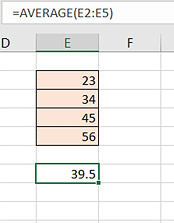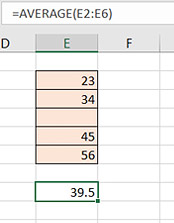Notice that in the first picture, the range (shaded pink) is filled with numbers. But in the second picture, there is a blank cell. However, the answer to both formulas is the same. The AVERAGE does not count how many cells, it counts how many numbers.

#### COUNTIF

Here's another function:

COUNTIF will find how many times a certain number or text string appears in a range. Let's say you have 25 cells filled with numbers, and you want to find out how many times a certain number appears. COUNTIF will find it.

COUNTIF needs two arguments: the first argument is where to search, and the second argument is what to search for. Remember, each argument is separated by a comma. So you would have the range reference B2:F6 first, then a comma, then the thing to search for:

=COUNTIF(B2:F6,5)The red portion is the range, and the blue area is what to search for

Here is what it might look like in Excel: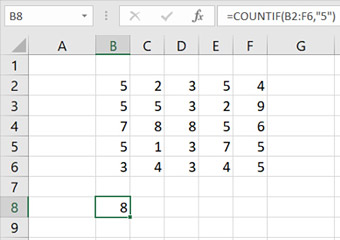Notice that this formula has quotes around the number. For a number, that is not necessary. It works with or without quotes.

You can also use COUNTIF to look for text strings, or words: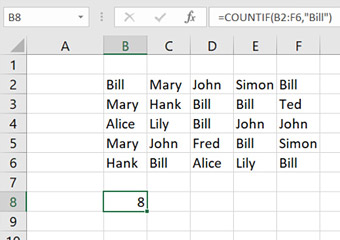In this case, we are looking for text; quote marks are necessary!

#### Other Functions

Excel has over 200 other functions. Categories include Financial, Date & Time, Math, Statistical, Lookup & Reference, Database, Text, Logical, and Information. For example, RAND will give a random number; CONCATENATE will add together any number of text strings; ROUND will change a decimal into a whole number; and so on. To see a whole list with explanations for each one, go to the INSERT menu and select the "Function..." command.

#### Fill

Now let's learn a very useful tool: the FILL feature.

When you select a cell, notice the outline: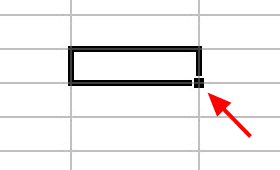Notice the little box at the lower right corner. This is called the fill handle. You can use it to fill other cells.

When your cursor is over normal cells, it is a fat, white plus-sign. But when you put the cursor over the fill handle, it becomes a thin, black plus-sign: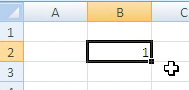When you have the thin, black plus-sign, you can click and drag. This will fill the new cells: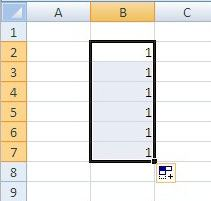So filling new cells will repeat the original cell. This works with numbers, text, or anything in a cell. Try typing any words or numbers and filling them.

#### Filling Patterns

You can also create special fill patterns. This time, type "1" in one cell, and then below it, type "2", like this: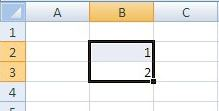Select both cells. You will see the fill handle at the bottom right of the lower cell. Click and drag the fill handle, and see what it makes: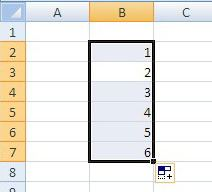You can see that it did not just copy the two cells! Instead, it noticed a pattern: 1, 2. It then decided to continue the pattern: 1, 2, 3, 4, 5, 6.

Try this with other numbers: "2, 4" for example. It will fill into "2, 4, 6, 8, 10".

Be careful, though: the FILL will only take the difference between the first two numbers. For example, if you type "1, 10" then Excel will NOT fill it to "1, 10, 100, 1000". Instead, it will fill it to "1, 10, 19, 28". Why? Because the difference between 1 and 10 is 9. So it will keep adding 9 to each new number.

Now try typing days and months--for example, "Monday" or "June". If you are on an English-language MS Word, it will fill the other days or months. If you are on a Japanese-language MS Word, try "月曜日" or "六月" and it should work.

You can make custom lists by going into the TOOLS menu and selecting OPTIONS. Select the CUSTOM LISTS tab. You will see the days and months lists here. You can use this to make your own special lists, if you like.

#### Filling Formulas

The FILL feature is most useful for repeating formulas.

Let's say you have three columns of numbers: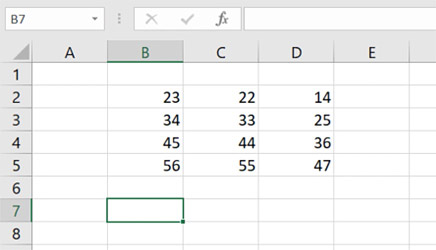Now make a formula to add the numbers in the first column: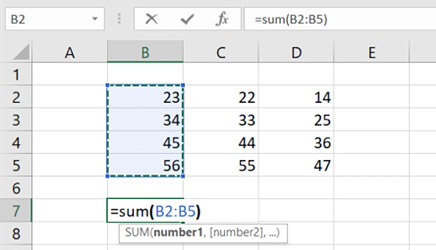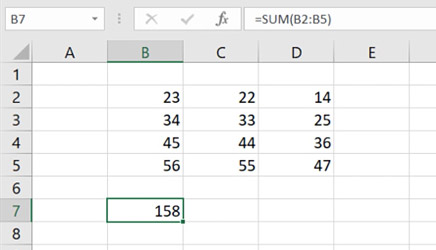But now, you want to add the numbers of the other two columns. Usually, you would type a new formula into each one. However, if you FILL the first formula from the left to the right: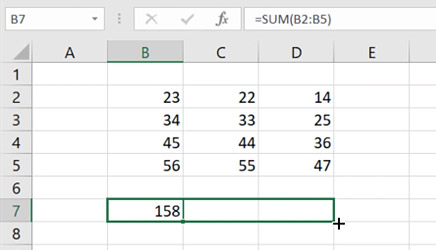It will copy the FORMULA into the new cells!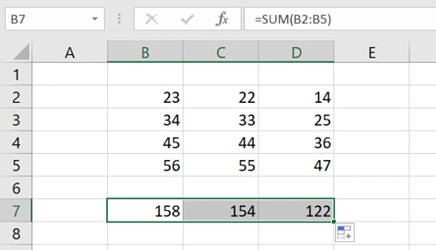That will give you the sum of the numbers in the other two columns!

This is a quick and easy way to repeat formulas when you have many identical calculations. The Formula Fill can be done left-and-right, or up-and-down.

### Terms to Know

functiona pre-made set of calculations you can carry out by using the function name in a formula
argumentone or more variables or data necessary to carry out a function
SUMa function to add values or else carry out a mathematical calculation
AVERAGEa function to find the average of a set of values
COUNTIFa function to find the number of times a value appears within a range
RANDa function to produce a random number
CONCATENATEa function to create a long string from more than one values
ROUNDa function to change a decimal into a number with fewer decimal places
fillan action which can repeat the contents of one cell, or to create a list based upon a pattern found in one or more cells
fill handlethe part of a selected cell which allows you to create a fill
Previous Chapter Chapter Practice Next Chapter